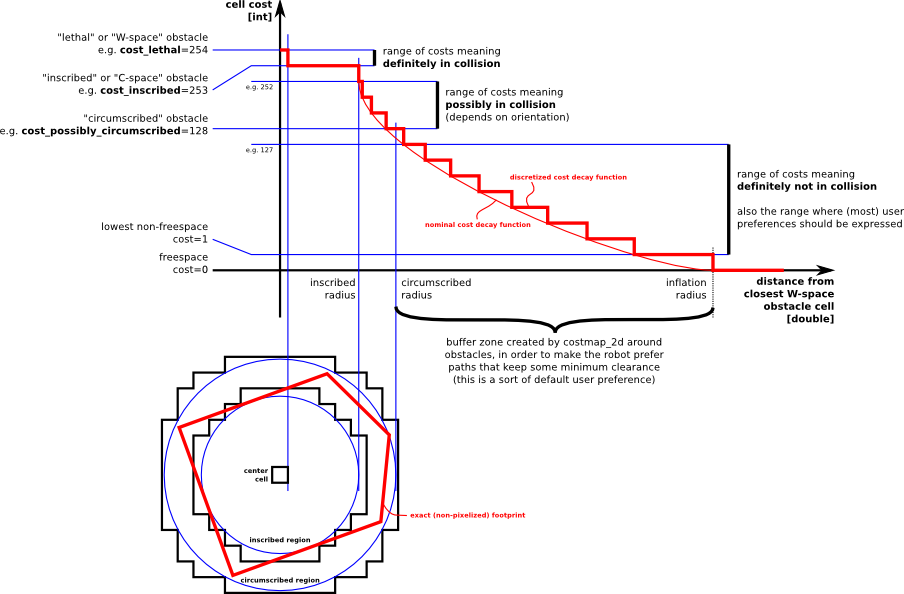[Documentation] [TitleIndex] [WordIndex]

Inflation Costmap Plugin

InflationInflation is the process of propagating cost values out from occupied cells that decrease with distance. For this purpose, we define 5 specific symbols for costmap values as they relate to a robot.

• "Lethal" cost means that there is an actual (workspace) obstacle in a cell. So if the robot's center were in that cell, the robot would obviously be in collision.
• "Inscribed" cost means that a cell is less than the robot's inscribed radius away from an actual obstacle. So the robot is certainly in collision with some obstacle if the robot center is in a cell that is at or above the inscribed cost.
• "Possibly circumscribed" cost is similar to "inscribed", but using the robot's circumscribed radius as cutoff distance. Thus, if the robot center lies in a cell at or above this value, then it depends on the orientation of the robot whether it collides with an obstacle or not. We use the term "possibly" because it might be that it is not really an obstacle cell, but some user-preference, that put that particular cost value into the map. For example, if a user wants to express that a robot should attempt to avoid a particular area of a building, they may inset their own costs into the costmap for that region independent of any obstacles.
• "Freespace" cost is assumed to be zero, and it means that there is nothing that should keep the robot from going there.
• "Unknown" cost means there is no information about a given cell. The user of the costmap can interpret this as they see fit.
• All other costs are assigned a value between "Freespace" and "Possibly circumscribed" depending on their distance from a "Lethal" cell and the decay function provided by the user.

The rationale behind these definitions is that we leave it up to planner implementations to care or not about the exact footprint, yet give them enough information that they can incur the cost of tracing out the footprint only in situations where the orientation actually matters.

ROS API

Parameters

~<name>/inflation_radius (double, default: 0.55)

• The radius in meters to which the map inflates obstacle cost values.
~<name>/cost_scaling_factor (double, default: 10.0)
• A scaling factor to apply to cost values during inflation. The cost function is computed as follows for all cells in the costmap further than the inscribed radius distance and closer than the inflation radius distance away from an actual obstacle: exp(-1.0 * cost_scaling_factor * (distance_from_obstacle - inscribed_radius)) * (costmap_2d::INSCRIBED_INFLATED_OBSTACLE - 1), where costmap_2d::INSCRIBED_INFLATED_OBSTACLE is currently 254. NOTE: since the cost_scaling_factor is multiplied by a negative in the formula, increasing the factor will decrease the resulting cost values.

2019-10-12 12:32